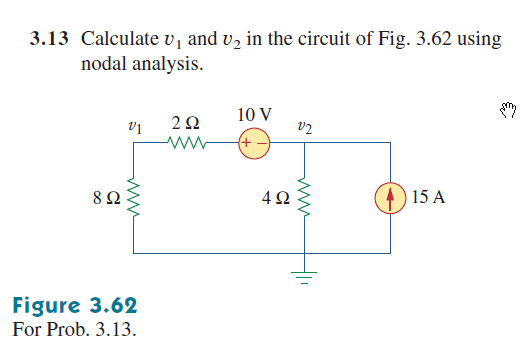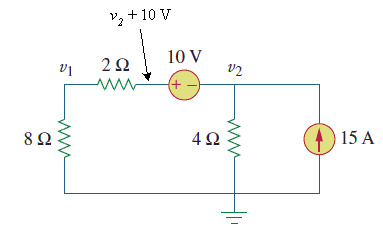# Finding Voltages in a Circuit with Nodal Analysis

## Homework StatementKCL: Σi = 0
KVL: Σv = 0

## The Attempt at a Solution

Using KCL:

15 = (v1)/8 + (v2)/4

Using KVL:

(v1) - (v2) = (I2)R + 10 (v1) - (v2) = (I2)2 + 10

(I2) = (v1)/8

(v1) - (v2) = (2)((v1)/8) + 10 (v1) - (v2) = (v1)/4 + 10

Now there are two variables and two equations.

Solve second equation for v1. (8)(15) = v1 + 2(v2) v1 = 120 -2(v2) Plug into first equation.

120 -2(v2) -v2 = (120 -2(v2))/4 + 10

120 - 3(v2) = 30 -.5(v2) + 10

120 -2.5(v2) = 40

-2.5(v2) = -80

v2 = 32

This could then be plugged back into either of the first equations to solve for v1. However, according to the solution in the back of the textbook, v2 = 40V not 32. What have I done wrong?

gneill
Mentor
I'm not sure where your algebra has gone off track, but I will say approach for finding the circuit equations is not a standard one for nodal analysis.

In nodal analysis you take one node at a time and write equations for the sum of the currents there, setting it to zero. You don't introduce new current variables (and then eliminate them with further gyrations).

So for example, at the v1 node you would write:

##\frac{v1}{8} + \frac{v1 - (v2 + 10)}{2} = 0##Note that each term in the above looks like an Ohms Law calculation for the current of a branch: potential difference over resistance. If you have a node with a known current flowing into or out of it (such as is the case for the v2 node) you simply sum that in as a separate term.

Can you write the node equation for the v2 node?

•Gwozdzilla
How did you determine the sign for (v2 + 10)? (Why isn't it (v2 - 10)? Is it just part of nodal analysis that when a voltage source is immediately next to one of the nodes, that voltage can be added to the node voltage and treated as a single node or "supernode"? Is this how a supernode is formed?

Here's my attempt at the equation at v2:

(v1 - (v2 + 10))/2 + (v2)/4 = 15

Is this correct or did I misplace a sign?

gneill
Mentor
How did you determine the sign for (v2 + 10)? (Why isn't it (v2 - 10)? Is it just part of nodal analysis that when a voltage source is immediately next to one of the nodes, that voltage can be added to the node voltage and treated as a single node or "supernode"? Is this how a supernode is formed?
Yup. That's that's what's behind supernodes. If you happen to have a branch with multiple voltage sources and resistances, you can sum them to yield a net voltage and net resistance for the branch.
Here's my attempt at the equation at v2:

(v1 - (v2 + 10))/2 + (v2)/4 = 15

Is this correct or did I misplace a sign?
While not mandatory, it is always best to write nodal equations as a sum equal to zero. That way you never have to think about how to juggle signs for currents when you move them from one side of the equation to the other. Just pick whether you are going consider all currents to be flowing in, or all currents flowing out. Then the current directions are selected by the way you write the Ohm's Law expression. So if you were writing a term for a current flowing from some node V1 to node V2 and the branch has resistance R, you write (V1 - V2)/R. If you are summing currents leaving node V2, then that same term would be written as (V2 - V1)/R. Note the order of the node voltages.

Once you get in the habit of doing it this way you can write the equations by inspection and not trip up over signs, because you do it the same way, every time. Personally I'm in the habit of always summing currents flowing out of a node. But it's your choice.

Now about your equation. Your first term is for a current flowing from node v1 into v2, but your second term is for a current flowing out of the node (to ground). Then the 15 being on the right is for a current flowing out of the node, even though the 15 A is flowing into the v2 node in the diagram. So you have a mixed bag of terms. Choose one direction (in or out) and sum accordingly.

If you happen to have a current source that flows into a node and you're summing currents flowing out of the node, then you change the sign of the term. That's really the only time you need to worry about signs: when a known current is flowing in the opposite direction of your in/out choice.

•Gwozdzilla
So it's okay to write all of the equations as if all of the currents are flowing out of every node even though that means changing sign conventions for the current through any given element?

Assuming that's okay, here's my second attempt:

((v2 + 10) - v1)/2 + (v2)/4 + 15 = 0

Since the equation is for the v2 node itself, I put the voltage (v2 + 10) as higher than v1, then I added all of the remaining currents.

gneill
Mentor
So it's okay to write all of the equations as if all of the currents are flowing out of every node even though that means changing sign conventions for the current through any given element?
Well, you're not changing a sign convention when you choose an assumed current direction for an unknown current. The node voltages and branch currents are unknowns. The exception when a current source is involved. Even so, a current source that is shown as flowing into a node with some value ##I## is identical to a current source flowing out of the node with a value ##-I##. It's just an algebraic manipulation.
Assuming that's okay, here's my second attempt:

((v2 + 10) - v1)/2 + (v2)/4 + 15 = 0

Since the equation is for the v2 node itself, I put the voltage (v2 + 10) as higher than v1, then I added all of the remaining currents.
Okay, the first term says the current is assumed to be flowing out of the node. That sets your assumed direction for the currents. The second term says the current is flowing out of the node. Good, that agrees with the chosen direction. But the third term says that 15 A is also flowing out of the node. That's a problem since the source in the diagram is flowing into the node. So in terms of your equation it should be -15 A flowing out (which is the same as saying +15 A flowing in).

•Gwozdzilla
Okay, so setting the current to be going into the node, while assuming that the other currents are flowing out of the node, my formula is this:

((v2 + 10) - v1)/2 + (v2)/4 - 15 = 0

Simplifying...

.5v2 + 5 - .5v1 + .25v2 - 15 = 0
.75v2 - .5v1 - 10 = 0
v2 = .667v1 - 13.3

Plugging this into the first equation:

(v1)/8 + (v1 - (.667v1 - 13.3 + 10))/2 = 0
v1 + 4v1 + 53.3 - 2.67v1 + 40 = 0
2.33v1 + 93.3 = 0
v1 = -40V
This is very close, but the solution in the back said that it was +40V. Is my formula still wrong?

gneill
Mentor
Okay, so setting the current to be going into the node, while assuming that the other currents are flowing out of the node, my formula is this:

((v2 + 10) - v1)/2 + (v2)/4 - 15 = 0

Simplifying...

.5v2 + 5 - .5v1 + .25v2 - 15 = 0
.75v2 - .5v1 - 10 = 0
v2 = .667v1 - 13.3 ← check the sign on the 13.3
When you move - .5v1 - 10 to the RHS, the signs of both terms must change.

•Gwozdzilla